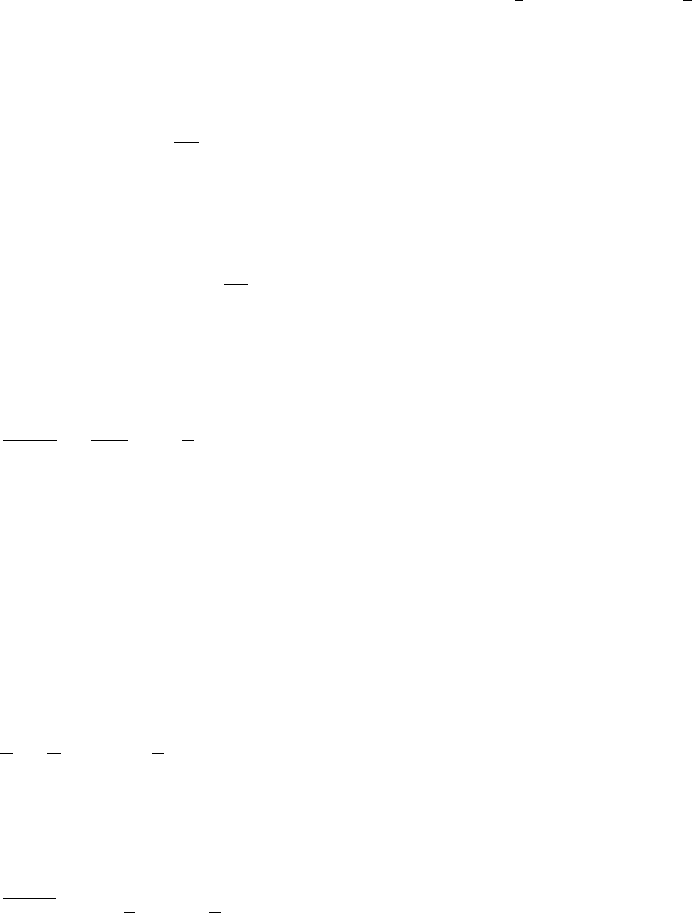# MATH137 Study Guide - Final Guide: Mean Value Theorem, Antiderivative

81 views4 pages
School
Department
Course
ProfessorMath 137 Sample Final 1 Answers
NOTE: Only answers are provided here. Remember that on the ﬁnal exam,
you MUST show all of your steps to get full marks
a) For every  > 0 there exists a δ > 0 such that f(x)<1
whenever x > 1
δ.
b) If f(x) is continuous on [a, b], then and F(x) is a function such that F0(x) = f(x) on
[a, b], then
d
dx Zx
a
f(t)dt =f(x),for x(a, b)
Zb
a
f(x)dx =F(b)F(a)
c) tan(sec1(4)) = tan(x) = 15.
2. a) lim
x3(x22x+ 1) = 4
b) lim
x1x
x11
ln x=1
2
3. a) f0(x) = exe1
b) g0(x) = sec(xsinh x) tan(xsinh x)(sinh x+xcosh x)
c) h0(x) = x2sin x
4. Evaluate the following integrals.
a) Z2
1x
22
xdx =3
42 ln 2
b) Z2
2|x|dx = 4
c) Zx2 + x dx =2
5u5/24
3u3/2+C
1
Unlock document

This preview shows page 1 of the document.
Unlock all 4 pages and 3 million more documents.

## Document Summary

 whenever x > 1 (cid:90) x (cid:90) b a d dx f (t) dt = f (x), for x (a, b) f (x) dx = f (b) f (a: tan(sec 1(4)) = tan(x) = a. 15. (x2 2x + 1) = 4 (cid:19: a) lim x 3 (cid:18) x, lim x 1 x 1. 2: a) f(cid:48)(x) = exe 1, g(cid:48)(x) = sec(x sinh x) tan(x sinh x)(sinh x + x cosh x, h(cid:48)(x) = x2 sin x, evaluate the following integrals. (cid:19) (cid:18) x. 1 x e2y = (cid:18) 1 + x (cid:19) (cid:18) 1 + x. Since lim x a that if 0 < |x a| < , then |f (x) l| < |c|. Thus, whenever 0 < |x a| < , we have f (x) = l, we get that for any  > 0, there exists a > 0 such. |cf (x) cl| = |c||f (x) l| < |c| .